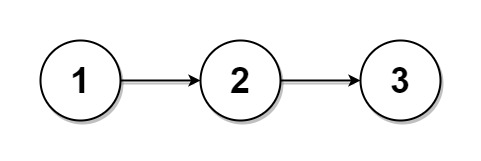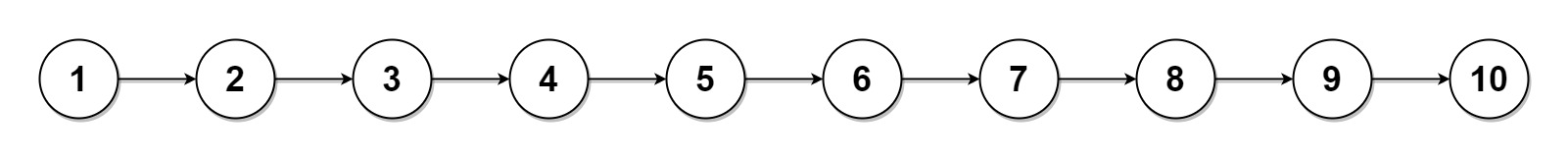# 725. Split Linked List in Parts

Medium
Given the `head` of a singly linked list and an integer `k`, split the linked list into `k` consecutive linked list parts.
The length of each part should be as equal as possible: no two parts should have a size differing by more than one. This may lead to some parts being null.
The parts should be in the order of occurrence in the input list, and parts occurring earlier should always have a size greater than or equal to parts occurring later.
Return an array of the `k` parts.
Example 1:Input: head = [1,2,3], k = 5
Output:
[,,,[],[]]
Explanation:
The first element output has output.val = 1, output.next = null.
The last element output is null, but its string representation as a ListNode is [].
Example 2:Input: head = [1,2,3,4,5,6,7,8,9,10], k = 3
Output:
[[1,2,3,4],[5,6,7],[8,9,10]]
Explanation:
The input has been split into consecutive parts with size difference at most 1, and earlier parts are a larger size than the later parts.
Constraints:
• The number of nodes in the list is in the range `[0, 1000]`.
• `0 <= Node.val <= 1000`
• `1 <= k <= 50`

### 解題

Runtime: 0 ms, faster than 100%
Memory Usage: 2.8 MB, less than 20%
/**
* type ListNode struct {
* Val int
* Next *ListNode
* }
*/
func splitListToParts(head *ListNode, k int) []*ListNode {
res := make([]*ListNode, k)
length := 0
for p != nil {
length++
p = p.Next
}
numNodes := int(math.Floor(float64(length) / float64(k)))
extraNodes := length % k
index := 0
n := numNodes
if extraNodes > 0 {
n++
extraNodes--
}
for pre != nil && cur != nil {
n--
if n == 0 {
res[index] = pre
pre = cur.Next
cur.Next = nil
cur = pre
index++
n = numNodes
if extraNodes > 0 {
n++
extraNodes--
}
} else {
cur = cur.Next
}
}
return res
}